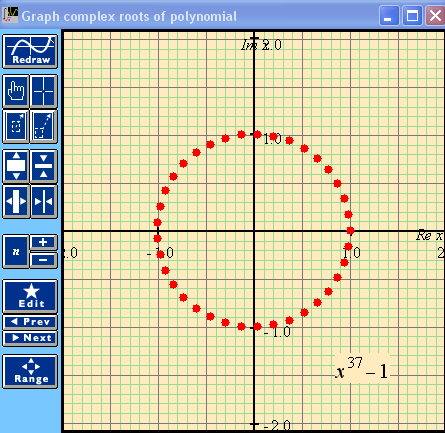# Help With Math: Purveyors of MathXpert

-->The Fundamental Theorem of Algebra asserts that every polynomial with complex coefficients has a root in the complex plane. MathXpert brings the visual realization of this theorem to the computer screen, showing all the roots of a complex polynomial. The example shows the "roots of unity", that is, the solutions of the equation xn=1. By clicking on the n button on the Graph Toolbar (in MathXpert, not here), you can rapidly change the value of n, thus demonstrating that all these roots lie on the unit circle and divide the circle into n equal parts. When this was first programmed, I asked my friend if he had ever seen such a graph before. "Only in my mind", he replied.